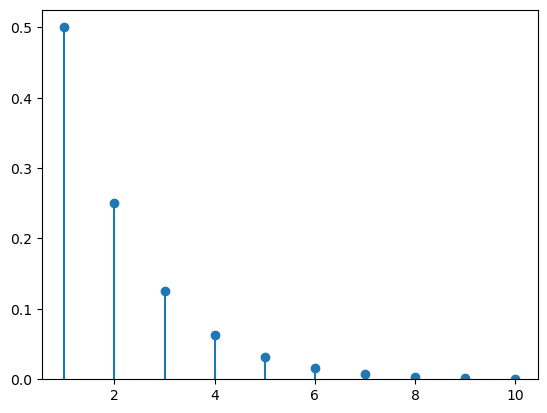# How to plot discrete probability distributions (in Python, using SciPy)

See all solutions.

There are many famous discrete probability distributions, such as the binomial and geometric distributions. How can we get access to them in software, to plot the distribution as a series of points?

## Solution

You can import many different random variables from SciPy’s stats module. The full list of them is online here.

The challenge with plotting a random variable is knowing the appropriate sample space, because some random variables have sample spaces of infinite width, which cannot be plotted.

The example below uses a geometric distribution, whose sample space is $\{1,2,3,\ldots\}$. We specify that we just want to use $x$ values in the set ${1,2,\ldots,10}$. (In some software, the geometric distribution’s sample space begins at 0, but not in SciPy.)

We style the plot below so that it is clear the sample space is discrete.

1
2
3
4
5
6
7
8
9
10
11
12
from scipy import stats
X = stats.geom( 0.5 )     # use a geometric distribution with p=0.5

import numpy as np
xs = np.arange( 1, 11 )   # specify the range to be 1,2,3,...,10

import matplotlib.pyplot as plt
ys = X.pmf( xs )          # compute the shape of the distribution
plt.plot( xs, ys, 'o' )   # plot circles...
plt.vlines( xs, 0, ys )   # ...and lines
plt.ylim( bottom=0 )      # ensure sensible bottom border
plt.show()